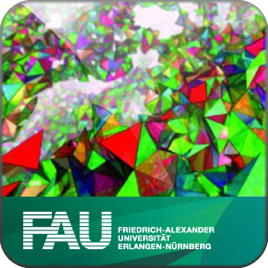20 episodes

Recently, the FAU Erlangen-Nuernberg initiated the Emerging Field Programme that supports research fields which have demonstrated a promising development but are just beginning to establish themselves in the scientific landscape. One of these "emerging fields" is "Quantum Geometry". In this Emerging Field Project, the expertise of both mathematicians and physicists are brought together in order to make progress in our understanding of Quantum Gravity. From the physics perspective, the task of combining the principles of General Relativity with those of Quantum Theory is one of the most important research problems in foundational physics. This requires novel mathematical input since the standard techniques of Quantum Field Theory fail in the case of the gravitational interaction. From the mathematical perspective, the new mathematical structures that arise in this effort may trigger progress in various mathematical disciplines such as infinite dimensional geometry, representation theory and related areas of Mathematical Physics. The interaction between mathematicians and physicists is therefore quite natural in this research field but but it poses a challenge due to the different backgrounds that these two research communities have. It is therefore the aim of this school to introduce the concepts and techniques of the respective research programme in a "bilingual" form from which both mathematicians and physicists can profit and can start interacting with each other.

The school will cover the following topics:

Group Valued Moment Maps
QFT on Curved Spacetimes
Loop Quantum Gravity
Infinite Dimensional Poisson Geometry

Lecturers

Anton Alekseev (Geneva U.)
Klaus Fredenhagen (Hamburg U.)
Jerzy Lewandowski (Warsaw U.)
Tudor Ratiu (EPFL, Lausanne)
Pavol Severa (Geneva U.)

Link to the Second Erlangen Fall School on Quantum Geometry:

http://www.video.uni-erlangen.de/course/id/232.html# First Erlangen Fall School on Quantum Geometry 2012/2013 (SD 640) Friedrich-Alexander-Universität Erlangen-Nürnberg

• Education

Recently, the FAU Erlangen-Nuernberg initiated the Emerging Field Programme that supports research fields which have demonstrated a promising development but are just beginning to establish themselves in the scientific landscape. One of these "emerging fields" is "Quantum Geometry". In this Emerging Field Project, the expertise of both mathematicians and physicists are brought together in order to make progress in our understanding of Quantum Gravity. From the physics perspective, the task of combining the principles of General Relativity with those of Quantum Theory is one of the most important research problems in foundational physics. This requires novel mathematical input since the standard techniques of Quantum Field Theory fail in the case of the gravitational interaction. From the mathematical perspective, the new mathematical structures that arise in this effort may trigger progress in various mathematical disciplines such as infinite dimensional geometry, representation theory and related areas of Mathematical Physics. The interaction between mathematicians and physicists is therefore quite natural in this research field but but it poses a challenge due to the different backgrounds that these two research communities have. It is therefore the aim of this school to introduce the concepts and techniques of the respective research programme in a "bilingual" form from which both mathematicians and physicists can profit and can start interacting with each other.

The school will cover the following topics:

Group Valued Moment Maps
QFT on Curved Spacetimes
Loop Quantum Gravity
Infinite Dimensional Poisson Geometry

Lecturers

Anton Alekseev (Geneva U.)
Klaus Fredenhagen (Hamburg U.)
Jerzy Lewandowski (Warsaw U.)
Tudor Ratiu (EPFL, Lausanne)
Pavol Severa (Geneva U.)

Link to the Second Erlangen Fall School on Quantum Geometry:

http://www.video.uni-erlangen.de/course/id/232.html

• video
1 - Infinite dimensional Poisson geometry - 01 2012/2013

## 1 - Infinite dimensional Poisson geometry - 01 2012/2013

Infinite dimensional Poisson geometry - 01

• 1 hr 23 min
• video
2 - Loop Quantum Gravity - 01 2012/2013

## 2 - Loop Quantum Gravity - 01 2012/2013

Loop Quantum Gravity - 01

• 1 hr 29 min
• video
3 - Infinite dimensional Poisson geometry - 02 2012/2013

## 3 - Infinite dimensional Poisson geometry - 02 2012/2013

Infinite dimensional Poisson geometry - 02

• 1 hr 25 min
• video
4 - QFT on curved spacetimes - 01 2012/2013

## 4 - QFT on curved spacetimes - 01 2012/2013

QFT on curved spacetimes - 01

• 1 hr 30 min
• video
5 - Loop Quantum Gravity - 02 2012/2013

## 5 - Loop Quantum Gravity - 02 2012/2013

Loop Quantum Gravity - 02

• 1 hr 28 min
• video
6 - Infinite dimensional Poisson geometry - 03 2012/2013

## 6 - Infinite dimensional Poisson geometry - 03 2012/2013

Infinite dimensional Poisson geometry - 03

• 1 hr 16 min